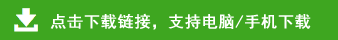# 苏教版三年级数学上册期末试卷

苏教版三年级数学上册期末试卷

1.直接写出得数。
12×5=      700×6=      27＋23=       65÷5=
42÷3=      60－24=      120÷3=       7×13=
4×18=      8×50=       400＋700=     15×6=
56÷4=      720÷8=      87÷3=        1200－600=